# ISEE Upper Level Quantitative : How to find mean

## Example Questions

1 2 4 Next →

### Example Question #123 : Data Analysis

In the first round of a gymnastics competition, six judges scored Rhonda's routine as follows: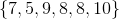In the second round, eight judges scored her routine as follows: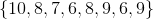In each round, the highest and lowest scores are removed to account for possible bias on the part of the judges, and each gymnast is awarded the mean of the remaining scores.

Which is the greater quantity?

(A) Rhonda's first round score

(B) Rhonda's second round score

It is impossible to determine which is greater from the information given

(A) and (B) are equal

(A) is greater

(B) is greater

(A) is greater

Explanation:

Rhonda's highest and lowest scores in the first round were 10 and 5, so her score in the first round was the mean of the scores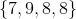.

This is the sum of the four scores divided by four, or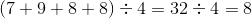.

Rhonda's highest and lowest scores in the second round were 10 and 6, so her score in the first round was the mean of the scores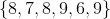.

This is the sum of the six scores divided by six, or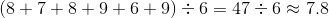.

(A) is greater.

### Example Question #32 : Mean

What is the average of the three numbers in the set below?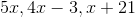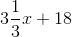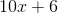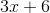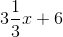Explanation:

The average is calculated by adding together the numbers in a set and dividing by the number of items: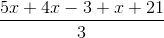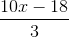### Example Question #124 : Data Analysis

The course average for a chemistry class is the mean of five test scores. Anne has scores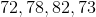so far; Barb has scores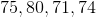so far. Which is the greater quantity?

(a) The score Anne must make to average(b) The score Barb must make to average(a) is greater.

(a) and (b) are equal.

It is impossible to tell from the information given.

(b) is greater.

(b) is greater.

Explanation:

The only real comparison that needs to be made is between the two students' totals; the one with the lesser total needs a greater score to average.

(a) Anne's total: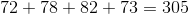(b) Barb's total: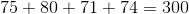Barb has fewer points so she needs more points to average. This makes (b) greater.

### Example Question #34 : Mean

Consider the following data set: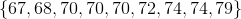Which of these numbers is greater than the others?

The mode of the set

It cannot be determined from the information given

The median of the set

The midrange of the set

The mean of the set

The midrange of the set

Explanation:

The median of the set is the fifth-highest value, which is; this is also the mode, being the most commonly occurring element.

The mean is the sum of the elements divided by the number of them. This is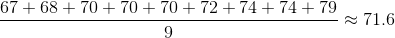The midrange is the mean of the least and greatest elements, This is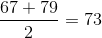The midrange is the greatest of the four.

1 2 4 Next →